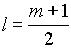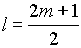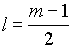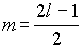SCHOLASTIC APTITUDE TEST 1995

CHEMISTRY

 Time: 1 Hour Max.Marks : 60

• Instructions:
• (1) Answer must be written either in English or the medium of instruction of the candidate in high school.

(2) There will be no negative marking

(3) There are FIVE Sections. Answer all questions of a section at one place

(4) Use of calculators or graph papers is not permitted

(5) Marks allotted for individual sections are as follows. All questions in an individual section carry equal marks.

SECTION-I

Differentiate the following: (10x2=20 Marks)

1. Equilibrium constant - Rate constant

2. Ionic product - Solubility product

3. Activation energy - Threshold energy

4. Valency - Oxidation state

5. Allotropy - Enantiotropy

6. Lattice energy - Hydration energy

7. Specific heat - Atomic heat

8. Electrolysis - Hydrolysis

9. Gangue - Slag

10. Diffusion - Effusion

SECTION-II

Explain the following Facts: (10x1=10 Marks)

1. White phosphorus is highly reactive than all other allotropic forms forms of phosphorus.

2. The bond angle is ammonia is 1070 but in CH4 is 1090 28’.

3. Aluminium containers are used to carry conc. HNO3.

4. The temperature of aqueous solution may increase or decrease when certain salts are dissolved.

5. Cl2 is non-polar while ICI is polar.

6. Electron affinity of nitrogen is zero while carbon and oxygen have substantial electron affinities.

7. NH3 is a base while NF3 is not a base.

8. Mercury acts as a conductor in the liquid state as well as in the solid state, whereas NaCl acts as a conductor only in the molten state.

9. Oxidising strength of halogens decrease down the group.

10. pH of the 10-8 M HCl is not equal to 8.

SECTION-III

Fill in the blanks: (15x1=15 Marks)

1. An element Z has two isotopes of atomic masses 16 and 18. Its overall atomic weight is 16.4. The proportion of the isotope 16 in Z is ______________________

2. The value of B is double that of `A’ and gas B is at double temperature and pressure. The number of moles B to A will be _______________________

3. 96.87% of a radio active substance disintegrated in 96.87 mints. The t1 of this is ___________

4. The total pressure of the mixture of two moles of oxygen and three moles of hydrogen is equal to 800 kpa. The partial pressure of oxygen in the mixture equals ______________ atm.

5. During the disintegration of radium, helium is produced. What volume of the helium produced at STP due to the disintegration of 2 grams of 224Ra after 3.65 days in a closed vessel. (t1 of 224Ra is 3.65 days) ______

6. The possible correct set of four quantum numbers for unpaired electron of chlorine atom _________

7. A gaseous oxide of nitrogen on strong heating gave a gaseous mixture not absorbed by water on cooling, the volume of gas found to be 1.5 times that of the oxide taken. Formula of oxide is _________

8. One mole of hydrogen iodide is heated in a closed container of capacity 2 litres. At equilibrium half a mole of hydrogen iodide has dissociated. The equilibrium constant is ___________

9. The number of sulphur atoms present in 100 ml of 0.1 M H2SO4 is _____________

10. A solution of sodium metal in liquid ammonia is strognly reducing due to the presence of __________.

11. When electron occupy orbitals of the same energy, each orbital is occupied by __________ with the spins of the electrons remaining ____________ before pairing takes place. This is principle is known as __________________

12. In the extraction of the copper from ore of copper, iron component is eliminated in the form of ________________ (formula)

13. Blue colour copper sulphate solution is stirred with Zn spoon, colour of the solution slowly fades away this is due to _________________________

14. The species M3+ has the electronic configuration 1s2 2s2 2p6 3s2 3p6 3d3. The atomic number of the element is _______________

15. Acid buffer solution of pH=3 is prepared by mixing sodium acetate and acetic acid. If the resulted solution is 10 times diluted pH of the solution is __________________.

SECTION-IV

Multiple Choice: (5x1=5 Marks)

1. The Khibini apatite contains 39.7% Ca, 38.1% O and 3.8% F the qualitative composition of the apatite can be represented by the formula

 A) Ca3(PO4)2F B) Ca5(PO4)F C) Ca5(PO4)3F D) Ca2PO4F

2. A solution with a mass percent of sodium hydroxide equal to 6% is prepared by adding the following mass of water (grm) to 200g of a solution with a mass percent of sodium hydroxide of 30%

 A) 300 B) 500 C) 800 D) 1000

3. KCl solution was left in a flask several weeks passed and a precipitate was formed in the flask. The solution over the precipitate is

 A) Dilute B) Super saturated C) Saturated D) Unsaturated

4. For a particular value of azimutal quantum number (l ) the total number of magnetic quantum number (m) is given by

 A)B)C)D)5. In the reaction between zinc and hydrochloric acid, a zinc cube of 1 gram mass was reduced into 1000 equal cubes. The volume of the evolving hydrogen per sec. will

 A) remains unchanged B) increases about 10 times C) increases by about 100 D) increases about 1000

SECTION-V

 Solve the Problems: (10 Marks)

1. What is the molecular weight of the substance, each molecule of which contains 8 carbon atoms, 13 hydrogen atoms and 3.33x10-23g of other components.

2. Radium disintegrates by emitting a particles. Rate of disintegration of radium is 8.15x1012 each second, during which 1.35x10-11 moles of helium is formed. Calculate Avagadro’s number.

3. Nitrogen, phosphorus, and potassium are plant nutrients that are provided for most crops in large quantities. Often chemicals containing these three nutrients are mixed in varied proportions to make fertilisers of different compositions. The composition of a given mixture is usually indicated by three numbers printed on the fertiliser bag. The first number is the percentage of nitrogen in the mixture; the second gives the amount of phosphorus as if it were present as P2O5 (that is, as the percentage of P2O5 in the mixture); the third gives the amount of potassium as if it were present as K2O in the mixture. For example, the numbers 15-10-5 indicate a composition of 15% total nitrogen, an amount of phosphorus equivalent to 10% P2O5, and an amount of potassium equivalent to 5% K2O.

Calculate the weights (in grams) of nitrogen, phosphorus, and potassium in a 1.00 kg sample of a 15-10-5 fertiliser. (Atomic weight of N=14, K=39, P=31)